## Abstract

To obtain optimum the power market the deregulation process is implemented for the entire electrical market and further classified as transmission and distribution units. Each firm focuses on delivering their best output which lead to congestion issues on transmission lines as there is in-adequate transmission capability to satisfy the load demand, transmission congestion occurs. Transmission cable congestion results from a lack of coordination between the utilities that handle generating, transmission, and distribution lead to generator failure, an abrupt increase in load demand, or the miscarriage of equipment, all of which can be fixed by FACTS devices. In this work, transmission line congestion is reduced using FACTS devices like UPFC and IPFC. The primary goal is to determine where FACTS devices should be placed. The main goal is to use the ANTLION optimization algorithm to locate the optimal position and size for FACTS devices. In an IEEE-14 bus system, UPFC and IPFC are individually connected and parameters are analyzed and formulated for better transmission among energy sectors.

Keywords: UPFC, IPFC, congestion, IEEE-14 bus system, ANTLION optimization

## 1. Introduction

Transmission line losses are due to the extreme loads, lack of co-ordination between generation, transmission and loads. There will be a loss of ability to retain power in the system as a result of an increase in distribution-side load and decreased power generation as discussed in paper . Further the proposed work establishes the goal of the study and identifies the characteristics of the system with and without FACTS devices identified in [2,3]. FACTS device play a major role to reduce power losses and improve the network's efficiency in order to minimize power loss . Different techniques have been discussed  to reduce power losses and maintain power quality; the most efficient topology and compact system method is used. The transmission line connects FACTS devices like the UPFC and IPFC. A comparison of UPFC and IPFC analyzed [6,7] and utilized to make up for the system's lagging output voltage by computing the difference between the reference voltage and output voltage. By using the FACTS device, the voltage difference is injected. An optimization technique called Antlion is used to determine the required size of the FACTS device as proposed in [8,9]. To obtain better power fed load as objective for FACTS device the proposed work algorithm analyzed the system with a FACTS device and the system without a FACTS device, an IEEE-14 bus test system is used to develop the model and presented for work.

## 2. Material and methods

One of the FACTS components, the unified power flow controller (UPFC), is connected in both a shunt and series form. Two back-to-back converters are included in the UPFC, converters 1 and 2, which are connected by a typical dc coupling provided by a dc storage capacitor. According to this theory, actual power can flow between the ac terminals of the two converters in any direction, and each converter can independently generate or absorb reactive power at its own ac output terminal. When a voltage sag or swell occurs in the transmission line, UPFC absorbs or generates reactive power to help maintain a constant output voltage. Converters 1 and 2 are coupled to the transmission line in series and shunt, respectively. ANTLION optimization algorithm is used to determine the optimal size and position of UPFC. The proposed block diagram of UPFC is shown below Figure 1.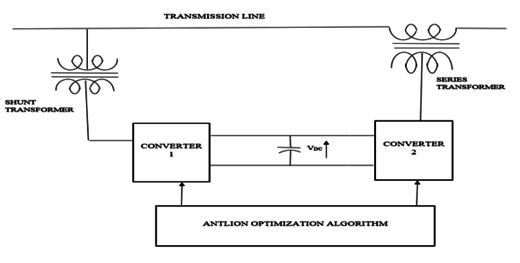Figure 1. Proposed block diagram of UPFC

One of the FACTS devices, the Interline Power Flow Controller (IPFC), is used to regulate the power flow of several transmission lines.  The device IPFC, which is derived from UPFC, contains n converters that regulate the power flow between the lines; the minimum number of converters that must be connected back to back is two. To manage the power flow, each converter must be connected in series with a distinct transmission line. In order to maintain control over the DC link voltage during this operation, active power must be coordinated. Each converter's reactive power is independent; the DC link voltage is achieved by similar way as UPFC. One series converter can produce an active power flow flowing into the link and can control voltage freely. The remaining n number of transmission lines transfer active power from one line to another to balance the power flow in the n parallel transmission lines if there are n number of converters and (n-1) converters can absorb or generate active power while one converter must control the DC link voltage. The ANTLION optimization algorithm is used to determine the optimal size and position of IPFC. The proposed block diagram of IPFC is shown below Figure 2.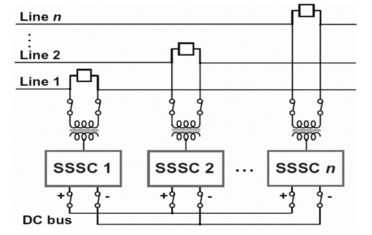Figure 2. Proposed block diagram of IPFC

### 2.1 ANT LION optimization algorithm

The majority of problems require precise values and strong tools for computing those values. Various methods are utilized for problem solving, and the ANTLION'S hunting skill aids in pinpointing the food. To search ants, ant lion makes use of the upcoming process. The proportionate value of each ANTLION is computed and placed in the matrices, and the position of the ants is saved there:

 $r(t)=\left\{{\begin{matrix}1\,rand>0.5\\0\,rand\,\leq 0.5\end{matrix}}\right.$
(1)

$Y(t)=[0,{\hbox{cumulative sum}}(2r(t_{1})-1)$, ${\hbox{cumulative sum}}(2r(t_{2})-1),\ldots$, ${\hbox{cumulative sum}}(2r(t_{n})-1),]$. Each antlion's proportionate value is calculated and recorded in the matrices:

 $\left|{\begin{matrix}f([A1.1\,,\,A1.2\,A1.3&\ldots &A1.d])\\f([A2.1\,,A2.2\,A2.3&\ldots &A2.d])\\\ldots \ldots \\f([An.1\,,\,An.2\,An.3&\ldots &An.d])\end{matrix}}\right|$
(2)

### 2.2 Pseudo code of ANT LION optimization algorithm

In a series of steps, ants move about randomly during an optimization process. The roulette wheel is used to construct traps. The manager of the roulette wheel chooses the lions based on fitness.

Step 1: Simulate the IEEE-14 bus test system and run the Simulink model without implementing FACTS device.

Step 2: Analyze the active power, reactive power, input voltage, output voltage characteristics through scope without FACTS device for 100% load.

Step 3: Increase the load moderately by 25% then the 100% original load and analyse the active power, reactive power, input voltage, output voltage characteristics.

Step 4: Constantly increase the load moderately by 25% than the 125% of previous load and analyses the active power, reactive power, input voltage, output voltage characteristics.

Step 5: Constantly increase the load moderately by 25% than the 150% of previous load and analyze the active power, reactive power, input voltage, output voltage characteristics.

Step 6: Differentiate the voltage difference for the 100% load, 125% load, 150% load, 175% load and find the disturbances in active and reactive power.

Step 7: Design the IEEE-14 bus test system with UPFC.

Step 8: Switch on the UPFC in the transmission line and apply the antlion algorithm in the simulation.

Step 9: Repeat the step 2 to step 6.

Step 10: The lagging voltage is improved by generating reactive power for the corresponding change in the load by using UPFC, the output voltage is improved nearly to the input voltage, the deviations in the real and reactive power characteristics are also improved for the corresponding change in the consecutive loads.

Step 11: Design the IEEE-14 bus test system with IPFC.

Step 12: Switch on the IPFC in the transmission line and apply the antlion algorithm in the simulation.

Step 13: Repeat the step 2 to step 6.

Step 14: The lagging voltage is improved by generating reactive power for the corresponding change in the load by using IPFC, the output voltage is improved nearly to the input voltage, the deviations in the real and reactive power characteristics are also improved for the corresponding change in the consecutive loads

Step 15: Compare the output voltage, real power, reactive power and voltage injected by UPFC and IPFC and find the best FACTS device which is suitable for the operation.

## 3. Results and discussion

In this work, the IEEE-14 bus test system is designed and analyzed using MATLAB & Simulink models shown in Figure 3 represent the proposed system. The system is connected along with UPFC and an additional switch is provided to know the characteristics of the system without IPFC and with IPFC. To turn on IPFC, 1 is given to run the system with IPFC and 0 is given, IPFC is in off state and run the system without IPFC. One generating bus is given and thirteen load buses are designed in this simulation.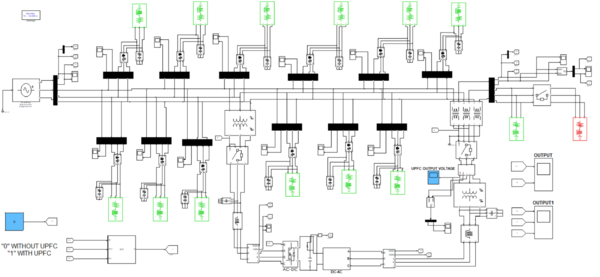Figure 3. Proposed simulation diagram of the system with UPFC

Figure 4 shows the real and reactive power of the proposed system without UPFC, there is no constant output is monitored and there are deviations in curves less than 0.05 seconds, and the proposed system, run with the base load of 10MW and we can see that the output voltage is lagging than the permissible voltage variation.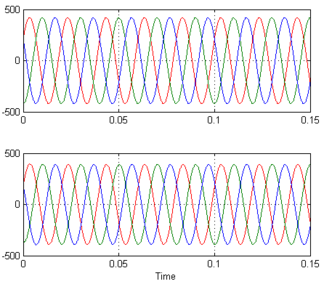Figure 4. Input and output voltage characteristics for 10MW load without UPFC

Figure 5 shows the lagging voltage is injected by UPFC, the algorithm monitors the input and output voltage and the voltage difference between the recommended voltage and the output voltage is monitored and according to the voltage difference, the UPFC inject voltage to compensate the lagging voltage. Figure 6 shows the real and reactive power deviations are minimized, compared to the system without UPFC and holds better the input and output voltage is compensated using UPFC and output voltage is improved nearer to input voltage.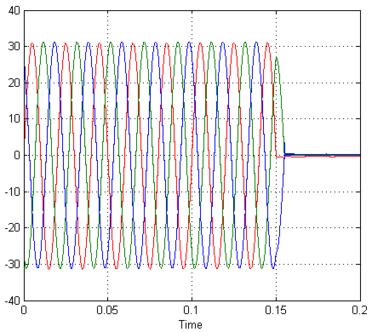Figure 5. Voltage injected by UPFC for 10MW load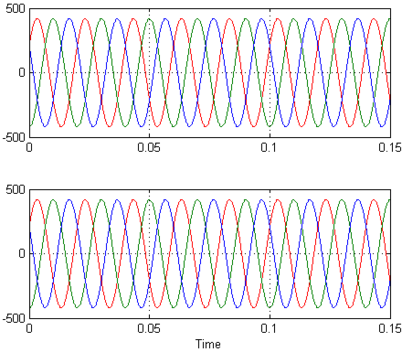Figure 6. Input and output voltage characteristics for 10MW load with UPFC

Table 1 contains the values of graphs that are obtained while the system works with UPFC and without UPFC. Figure 7 shows the real and reactive power of the proposed system without IPFC, there is no constant output is monitored and there are deviations in curves less than 0.05 seconds. Figure 8 shows the proposed system, run with the base load of 10MW and we can see that the output voltage is lagging than the permissible voltage variation.

Table 1. Numerical representation of the simulated graph
in percentage
in MW
Input voltage
(VOLTS)
Output voltage
(VOLTS)
Lagging voltage
(VOLTS)
Output voltage after placing UPFC
(VOLTS)
100 10 415 385 30 415
125 12.5 415 376 39 415
150 15 415 370 45 415
175 17.5 415 360 55 415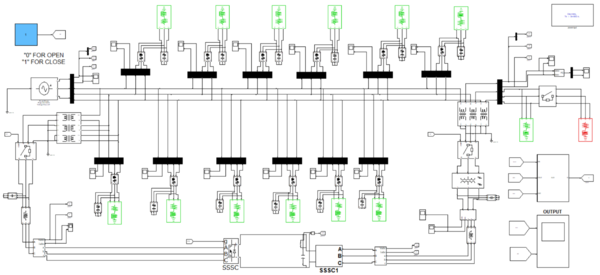Figure 7. Proposed simulation diagram of the system with IPFC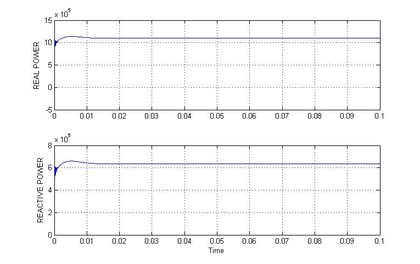Figure 8. Real and reactive power characteristics for the load 10MW load without IPFC

Table 2 contains the value of graphs that are obtained while the system works with IPFC and without IPFC. Figure 9 shows the lagging voltage is injected by IPFC, the algorithm monitors the input and output voltage and the voltage difference between the recommended voltage and the output voltage is monitored and according to the voltage difference, the IPFC inject voltage to compensate the lagging voltage. Figure 10 shows the real and reactive power deviations are minimized, compared to the system without IPFC. Figure 11 shows the input and output voltage is compensated using IPFC and output voltage is improved nearer to input voltage.

Table 2. Numerical representation of the simulated graph
in percentage
in MW
Input voltage
(VOLTS)
Output voltage
(VOLTS)
Lagging voltage
(VOLTS)
Output voltage after placing UPFC
(VOLTS)
100 10 415 384 31 415
125 12.5 415 376 39 415
150 15 415 369 46 415
175 17.5 415 361 54 415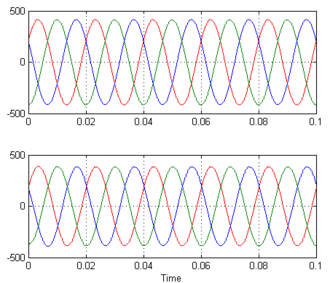Figure 9. Input and output voltage characteristics for 10MW load without IPFC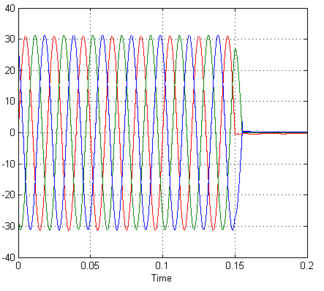Figure 10. Lagged voltage injected by IPFC for 10MW load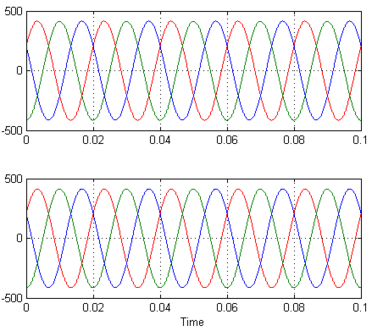Figure 11. Input and output voltage characteristics for 10MW load with IPFC

## 4. Conclusion

In this work, congestion management was done by using FACTS device. The IEEE-14 Bus test system has been designed in MATLAB/Simulink and it is simulated with the system connected with FACTS device and without FACTS device. Simulation results show the FACTS device effectiveness by injecting the required voltage need by the system to maintain balance output voltage and it is found that the active and reactive power is also improved when FACTS device is introduced. The optimal allocation of FACTS device is done by using ALO algorithm. Moreover, UPFC and IPFC inject the required amount of voltage to maintain the system output voltage at constant. During the voltage injection, whereas IPFC injects only the required amount of voltage to the load but UPFC injects high voltage at the initial stage. To protect the system from damage, occur due to high voltage. The system needs to add protection circuit, which adds on additional cost on the system. This main of this work is to reduce cost and to increase the efficiency of the system. So, IPFC is comparatively better than UPFC to improve the efficiency of the system.

### Document informationPublished on 20/06/23
Accepted on 10/06/23
Submitted on 03/10/22

Volume 39, Issue 2, 2023
DOI: 10.23967/j.rimni.2023.06.003

### Document Score0

Views 29
Recommendations 0